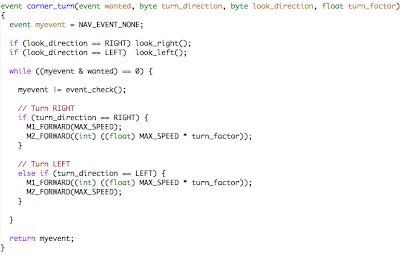## Thursday, June 19, 2008

### Pokey's Software: Part 3

Constant Radius Turns. Long overdue, here's more on Pokey's code. Pokey has no encoders and I kind of like the challenge of trying to navigate without them. One has to find ways to distill the analog world into simpler, discrete problems. We already talked about event based navigation as a way to do that. One can make certain decisions about how the robot should tackle the firefighting maze so that the robot is presented with a limited set of navigation/motion scenarios. For example:
1. Turn into room by making a u-turn around a wall
2. Turn into a room when you're at the end of a hallway
3. Turn a corner in the hallway
4. Follow a wall until something happens
5. etc.
One could set up the wall following to also handle turning around corners but there's two problems. Tuning wall following for aggressive correction means overly sharp turns around corners and spinning when too far from the wall (the firefighting walls are wide, Pokey isn't). More importantly, scenario #2 requires a turning routine independent of wall following anyway. I'd rather have one turning routine than two.

To solve the above scenarios it was easiest to stitch together a discrete set of motion behaviors, one of which is a constant radius turn. That way Pokey can follow the wall (another motion behavior) until it isn't there, and execute a constant radius turn around the corner. Each discrete motion can be independently tuned and life is happy. But how to make the robot trace a constant radius turn regardless of speed ... or battery charge?

I wondered if the ratio of the distances traveled by each wheel in a turn might also be the ratio of PWM signals applied to each motor. This assumes some things that I didn't want to noodle over. So I just tried it by varying pwm of one motor while setting the pwm of the other motor as a ratio of the first:

Close enough for government work as they say.

One can figure out the distance each wheel will travel on a turn of radius r, given a robot of track width w. Keeping it simple, measure r as the radius of the circle scribed by the inner wheel. Circumference, as every person on the street knows, is 2r * pi. So the outer wheel circle's circumference is 2(r+w) * pi.

Take the ratio of these, simplify algebraically (1+w/r), plug in w, and then you can either make a function that takes r as an argument and spits out the ratio, or manually start plugging in various values of r to build a table and make an interpolation/lookup table. Instead I just created a function for turning within nav.c where you supply the ratio (turn_factor) and I chose a hardcoded value when calling it from the main routine. Sloppy, but it worked. Incidentally the nav.c module holds all the lower level discrete behaviors: wall following, going straight, and turning.You give the routine what direction to turn (LEFT or RIGHT -- constants) and what direction to point the IR ranger array (LEFT or RIGHT again) -- look_direction. Then you tell it what events make it stop (wall found, floor mark found, etc.)

Ideally it would be more elegant to choose a wall following distance (maybe self calibrating by reading distance to wall at the start) and set the turn radius to maintain that distance. Also, to keep the average robot speed constant for smoother looking motion, figure out the radius to the center of the robot and vary both motor PWMs. If you simply reduce the inner motor's power, the robot slows down slightly on turns, but at least this looks more natural than speeding up around turns.